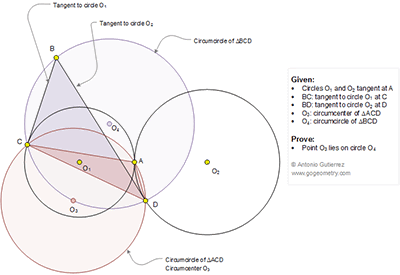## Tuesday, July 14, 2015

### Geometry Problem 1134: Tangent Circles, Tangent Line, Triangle, Circumcircle, Circumcenter

Level: Mathematics Education, High School, Honors Geometry, College.

Click the diagram below to enlarge it.#### 1 comment:

1.Points A ,O1 and O2 are collinear
Lets line joining A,O1 and O2 intersects BD at E and BC at F.
Lets assume angle AO2D = x
And angle AO1C = y
We can see that Angle BEF = 90 - x
And Angle BFE = y - 90
And we get Angle EBF = Angle DBC = 180 - (y-x)
Also Angle O1AC = 90 - y/2
Angle O1AD = 90 + (x/2)
We get angle DAC = 180 - [(y-x)/2]
Since O3 is circumcentre of triangle ACD
Angle DO3C = y - x
It means quad. BCO3D is cyclic and O3 lies on circle O4.